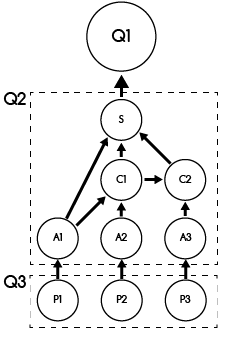# Query Processing¶

On this page, we describe how VerdictDB could speed up query processing. Note that for query processing, VerdictDB internally creates directed acyclic graph (DAG) representations (as described on this page) and use it for processing queries. The key to VerdictDB's faster query processing is how the DAG is constructed, which we describe below.

## DAG Construction¶

For description, we use the following example query:

```select product, avg(sales_price) as avg_price
from (
select product, price * (1 - discount) as sales_price
from sales_table
where order_date between date '2018-01-01' and date '2018-01-31'
) t
group by product
order by avg_price desc
```

In the above example, the inner query (projection) computes the price after discount, i.e., `sales_price`, and then the outer query (aggregation) computes the average of `sales_price`. Although this example query may be flattened into a simpler form, we intentionally use this nested form to make our description more general. Also, although VerdictDB internally parses the query (in String format) into its internal Java objects, our description will keep using the query string for easier understanding.

We suppose that a scramble `sales_table_scramble` has been created for the `sales_table` table, and `sales_table_scramble` contains three blocks. As described on this page, each block of the scramble amounts to a random sample of the original table, i.e., `sales_table`.

### Step 1: regular DAG construction¶

The given query is decomposed into multiple queries, each of which is a flat query. Except for the root node, all other nodes include `create table as select ...` queries. The query for a parent node depends on its children.

Below we depict the DAG and the queries for those nodes.```-- Q1
select *
from temp_table2
```
```-- Q2
create table temp_table2
select product, avg(sales_price) as avg_price
from temp_table1 t
group by product
order by avg_price desc
```
```-- Q3
create table temp_table1
select product, price * (1 - discount) as sales_price
from sales_table_scramble
where order_date between date '2018-01-01' and date '2018-01-31'
```

### Step 2: progressive aggregation DAG construction¶

VerdictDB converts a part of the DAG to enable progressive aggregations. The affected parts are the aggregate queries including scrambles in its from clause or the projections of scrambles. After the conversion, the DAG looks like below.First, each of the projection nodes at the bottom only involves a part of the scramble. In this simple example, the single scramble is split into three projections. See that the following query includes an extra filtering predicate, i.e., `verdictdbblock = 0`, to only select the particular block.

```-- P1
create table temp_table1
select product, price * (1 - discount) as sales_price
from sales_table_scramble
where order_date between date '2018-01-01' and date '2018-01-31'
and verdictdbblock = 0
```

Second, the aggregation is separately computed for each of those projections. It is important to note that the original `avg` function was converted into two separate aggregate functions, i.e., `sum` and `count`. The `avg` function value will be restored later.

```-- A1
create table temp_table2
select
product,
sum(sales_price) as sum_price,
count(sales_price) as count_price
from temp_table1 t
group by product;
```

Observe that the individual aggregation nodes (A1, A2, and A3) only involves its own verdictdbblock, i.e., identified with 0, 1, and 2. To compute the exact answers, we combine those individual aggregates using additional nodes Combiners (C1 and C2). Naturally, the number of the Combiners is always one fewer than the number of individual aggregate nodes. Suppose A1 creates a temporary table `temp_table2`, A2 creates `temp_table3`, and A3 creates `temp_table4`. Then, the Combiners perform the operations as follows.

```-- C1
create table temp_table5
select
product,
sum(sum_price) as sum_price,
count(count_price) as count_price
from (
select *
from temp_table2
union all
select *
from temp_table3) t
group by product;
```

The nodes also propagate some necessary metadata about the processed verdictdbblocks thus far.

Finally, the node S collects those aggregates, scale them appropriately, and restore the original select items.

```-- S
create table temp_table7
select product, (3.0 * sum_price) / (3.0 * count_price) as avg_price
from temp_table5
group by product;
```

In the above query (for the node S), `3.0 * sum_price` is an unbiased estimator for `sum(sales_price)`, and `3.0 * count_price` is an unbiased estimator for `count(sales_price)`. The dividing the sum by the count, we obtain the average. Note that those scaling factors differ depending on the source nodes (e.g., A1, C1, and C2).

### Step 3: plan simplification¶

VerdictDB simplifies the plan if possible. This process helps avoiding unnecessary temporary table creations.

### Step 4: Execution / Cleaning¶

VerdictDB executes the plan and removes the temporary tables if necessary.

## How Individual Aggregates Combined?¶

VerdictDB applies different rules for different types of aggregate functions as follows. VerdictDB relies on the state-of-the-art techniques available in the literature.

### AVG, SUM, COUNT¶

VerdictDB's answers are always unbiased estimators of the true answers. For instance, if only 10% of the data (that amounts to the 10% uniform random sample of the data) is processed, the unbiased estimator for the count function is 10 times the answer computed on the 10% of the data. This logic becomes more complex as unbiased samples (within scrambles) are used for different types of aggregate functions. VerdictDB performs these computations (and proper scaling) all automatically.

### MIN, MAX¶

VerdictDB's answers to min and max functions are the min and max of the data that has been processed so far. For example, if 10% of the data was processed, then VerdictDB outputs min or max among those 10% data. Of course, the answers become more accurate as more data is processed and become exact when 100% data is processed. One possible concern is that the answers based on partial data may not be very accurate especially when a tiny fraction (e.g., 0.1%) of the data has been processed. To overcome this, VerdictDB processes outliers first. As a result, even the answers at the early stages are highly accurate.

### COUNT-DISTINCT¶

This is in preparation.